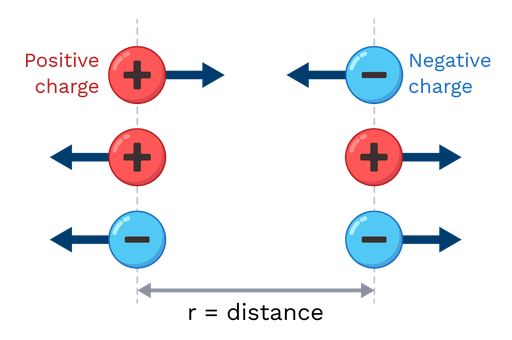Coulomb’s law is the law that determines the electrostatic force between two charged particles.

Coulomb’s law states that the magnitude of the force is directly proportional to the electrical charge of the particles and inversely proportional to the square of the distance between them.

In equation form:

Fe= k·|q1|·|q2|/r2.

Here, |q1| and |q2| are the amount of the electrical charge of the particles (taken as positive numbers), r is the distance between them, and k is a constant of proportionality. This equation determines only the strength of the force and not its direction.

Coulomb’s law states that the direction of the electrostatic force is determined by this rule: The force acts along the line joining the two charges and it is repelling for charges of the same sign, but attractive for charges of opposite signs. That is, the electrostatic force pulls together charges of opposite signs, but drives charges of the same sign apart.

Due to more advanced principles of electromagnetism, Coulomb's law can be applied to spheres of charge (not only particles) if distances are measured from their centers (not their surfaces).Figure: Charges of opposite signs attract, while charges of the same sign repel.